GAS COMPOSITION MEASUREMENT

BY HELMHOLTZ RESONATOR

N.M. Barlea,

Technical University of Cluj-Napoca, Physics Departement

Introduction

The gas composition measurement is an important aim both in the industrial processes and in the domestic area. Using the sound velocity for the gas composition measurement is not a new idea  but is an attractive one because of the low power consumption and to the fact it is direct related to the molar mass of the gas :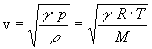(1)

where: g - adiabatic constant of the gas;
R - universal gas constant;
M - molar mass of the gas;
r - density of the gas;
T - temperature of the gas;
p - pressure of the gas.

The Helmholtz resonator consist of the elasticity of the gas closed in the volume V and the mass of the gas from the tube of section S and length l which links the volume V with the outer medium. Its resonance frequency is :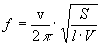(2)

We take advantage to the fact that the piezoelectric acoustic generators (buzzers), commercially available, are design to match the resonant frequency of the piezoelectric element with the resonant frequency of the case (Helmholtz resonator). The impedance of the device is minimum at the resonance.

The Experiment

The experimental arrangement consists of a test oscillator the piezoelectric acoustic generator in series with an AC digital ammeter (fig. 1). The acoustic generator is located into the test chamber, with the possibility to change the gas composition by injecting known volumes of methane with a syringe.

With a RMS voltage of 0.1V from the test oscillator, the measured currents through the piezoelectric acoustic generator in pure air at different frequencies are listed in Table 1.

TABLE 1
 Frequency (kHz) 3.8 3.9 4 4.03 4.1 4.13 4.2 Current (m A) 59.5 65.8 72.1 73.4 58.6 43.2 50.5

Maintaining a fixed frequency of 4,1 kHz and a RMS voltage of 0.1 V, we change the gas composition injecting methane (1ml steps) into the test chamber (180 ml volume). The measured currents versus the methane concentrations are listed in Table 2.

TABLE 2
 Methane conc. (%) 0 0.55 1.11 1.66 2.77 Current (m A) 58.6 59.6 60.5 61.5 63.3

From table 2 we have a current sensitivity to methane concentration of: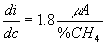(3)

and from the table 1 a current sensitivity to frequency change between 4.03 kHz and 4.1kHz of: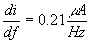(4)

From eq. (3) and (4) we can compute the frequency shift due to the gas concentration change being: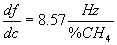(5)

Conclusions

From eqs. (1) and (2) we can calculate the relative frequency change due to the molar mass change: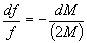(6)

Because the molar mass is related to the gas composition like: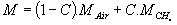(7)

and the resonant frequency change due to the gas concentration change is: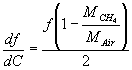(9)

We evaluate eq. (9) for MCH4 = 16g/mole, MAir = 28 g/mole, f = 4.03 kHz, dG = 1% and found :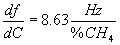(10)

a value very close to the experimental one from eq. (5).

The conclusion of this experiment is that Helmholtz resonator is a sensitive device for the gas composition measurement in the percent range of the binary mixtures of gas with different molar masses, like H2, CH4, CO2, propane or butane in air. It remains to solve the influence of the temperature gas detector.

References

 H. S. Shillingford, Brit. Commun. and Electronics, 1 (Jan. 1961), p. 26.

 M. W. Zemansky, R.H. Dittman, Heat and Thermodynamics (McGraw-Hill International book 1982) p. 120.

 D. Stanomir, Electroacoustic Systems (Ed. Tech. 1984, in roumanian), p. 189.